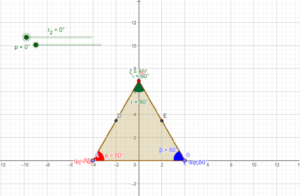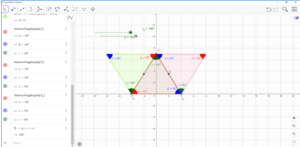# Angle sum property of a triangle using GeoGebra

A triangle has three sides and three angles, one at each vertex. The angle sum property of a triangle states that the sum of the angles of a triangle is equal to 180º. Whether a triangle is an acute, obtuse, or a right triangle, the sum of its interior angles is always 180º.

The angle sum property of a triangle is one of the most frequently used properties in geometry.

The angle sum property is used to find the measure of an unknown interior angle when the values of the other two angles are known.

Let’s see the practical execution of the angle sum property of the triangle using GeoGebra.

Step 1:

• Plot two points and draw a triangle using the polygon.
• Let’s name it ABC.
• Now find the midpoint of AC and BC by clicking on the midpoint or center icon.
• That midpoint is named point D.
• By using a slider operation, create an angle slider (r) with the following values.
• Set Min – 0 degree; Max- 180 degrees and increment as 10 degrees.
• Now by using rotate around point operation, click on point D and set the degree of rotation to slider r and set it as clockwise direction and click ok.• The same way create another slider operation for p and set the corresponding angle value as p.
• By using the Angle measure operation measure the angles and label and colour them accordingly.
• Give the input as the Sum of the angles and we can see the output as 180 degrees.
• Now the slider can be moved and the angle sum property can be observed.
• Even point C can be moved to observe the angle sum property for different angle combinations.